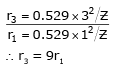Courses

# Competition Level Test: Structure of Atom- 2

## 30 Questions MCQ Test Chemistry for JEE | Competition Level Test: Structure of Atom- 2

Description
This mock test of Competition Level Test: Structure of Atom- 2 for Class 11 helps you for every Class 11 entrance exam. This contains 30 Multiple Choice Questions for Class 11 Competition Level Test: Structure of Atom- 2 (mcq) to study with solutions a complete question bank. The solved questions answers in this Competition Level Test: Structure of Atom- 2 quiz give you a good mix of easy questions and tough questions. Class 11 students definitely take this Competition Level Test: Structure of Atom- 2 exercise for a better result in the exam. You can find other Competition Level Test: Structure of Atom- 2 extra questions, long questions & short questions for Class 11 on EduRev as well by searching above.
QUESTION: 1

### Radiation of λ = 155 nm was irradiated on Li (work function = 5eV) plate. The stopping potential (in eV) is.

Solution:

3 eV
Corite Actual
Correct option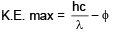= 8 – 5
= 3eV
∴ V0 = 3 eV

QUESTION: 2

### Increasing order of magnetic moment among the following species is __________ . Na+, Fe+3, Co2+, Cr+2

Solution:

Na+, Co+2, Cr2+, Fe+3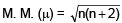we get Na+, Co+2, Cr2+, Fe+3

QUESTION: 3

### If in the hydrogen atom P.E. at ∞ is choosen to be 13.6 eV then the ratio of T.E. to K.E. for 1st orbit of H-atom is __________ .

Solution:

Zero
Refrence level is Ist orbit
itself T. E. = 0
Ratio becomes zero

QUESTION: 4

The light radiations with discrete quantities of energy are called ___________ .

Solution:

A photon is the quantum of electromagnetic radiation. The term quantum is the smallest elemental unit of a quantity, or the smallest discrete amount of something. Thus, one quantum of electromagnetic energy is called a photon. The plural of quantum is quanta.

QUESTION: 5

The ratio of the energy of a photon of 2000 Å wavelength radiation to that of 4000 Å radiation is

Solution: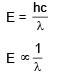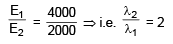QUESTION: 6

The energy of electron is maximum at :

Solution:

An electron bound to the nucleus by the mutual attraction between unlike charges, has a negative energy. Its most tightly bound to the nucleus nearer it is to the nucleus. That is an electron has least energy when it is in the n=1 shell. As the electron moves away from the nucleus its energy becomes less and less negative, i.e. its energy increases. When the electron is not under the attractive Coulomb force of the nucleus its potential energy is zero and whatever energy it has now, is kinetic energy, which is always positive. So farther the electron more energy it has.

QUESTION: 7

Which electronic level would allow the hydrogen atom to absorb a photon but not to emit a photon:

Solution:

Is → As it is the ground state

QUESTION: 8

The third line in Balmer series corresponds to an electronic transition between which Bohr's orbits in hydrogen :

Solution:

Balmer means transition to n = 2
1. line → 3 to 2
2. line → 4 to 2
3. line → 5 to 2

QUESTION: 9

The orbital angular momentum of an electron in 2s orbital is :

Solution:

Orbital Angular momentum is given by [√l(l+1)]×h/2π
For s orbital, we have l = 0
Therefore orbital angular momentum = 0

QUESTION: 10

Which quantum number is not related with Schrodinger equation :

Solution:

Spin quantum number do not follow Schrodinger Wave Equation because it is related to spin of the electrons.It is needed to explain Pauli's exclusion principle which sates that no two electrons can share the same state (i.e. have the same quantum numbers) at the same time.Therefore, if the quantum numbers n, l and are same then the electrons should have different spin.

QUESTION: 11

The shortest wavelength of He atom in Balmer series is x, then longest wavelength in the Paschene series of Li+2 is :

Solution: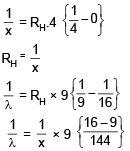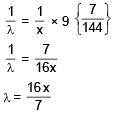QUESTION: 12

An electron in a hydrogen atom in its ground state absrobs energy equal to the ionisation energy of Li+2. The wavelength of the emitted electron is

Solution: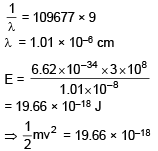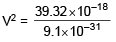V2 = 4.32 × 1013
V2 = 43.2 × 1012
V = 6.57 × 106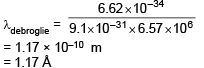QUESTION: 13

An electron, a proton and an alpha particle have kinetic energies of 16E, 4E and E respectively. What is the qualitative order of their de-Broglie wavelengths?

Solution:

Mass of a particle = 4 (mass of proton)
Mass of proton = 1840 (mass of e)
Let Mass of e = m
∴ Mass of p+ = 1840 m
and mass of a particle = 7360 m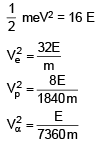QUESTION: 14

Given ΔH for the process Li(g) → Li+3(g) + 3e- is 19800 kJ/mole & IE1 for Li is 520 then IE2 & IE3 of Li+ are respectively (approx, value) :

Solution:

IE1 + IE2 + IE3 = 19800
IE2 + IE3 = 19800 – 520
IE2 + IE3 = 19280

QUESTION: 15

The ratio of difference in wavelengths of 1st and 2nd lines of Lyman series in H-like atom to difference in wavelength for 2nd and 3rd lines of same series is :

Solution: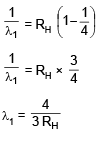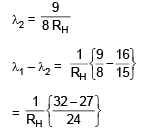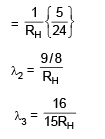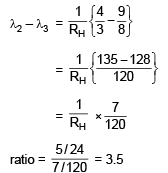QUESTION: 16

If radius of second stationary orbit (in Bohr's atom) is R. Then radius of third orbit will be

Solution: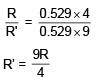= 2.25 R

QUESTION: 17

The ratio of wave length of photon corresponding to the α-line of lyman series in H-atom and β-line of Baimer series in He+ is

Solution: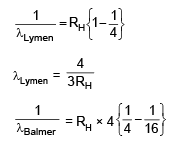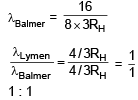QUESTION: 18

Three energy levels P, Q, R of a certain atom are such that EP < EQ < ER. If λ1, λ2, and λ3, are the wave length of radiation corresponding to transition R → Q : Q → P and R → P respectively. The correct relationship between λ1, λ2 and λ3 is

Solution:

We know that, for wave no.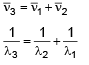QUESTION: 19

The Value of (n2 + n1) and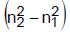for He+ ion in atomic spectrum are 4 and 8 respectively. The wavelength of emitted photon when electron jump from n2 to n1 is

Solution: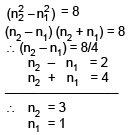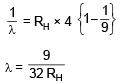QUESTION: 20

Number of possible spectral lines which may be emitted in bracket series in H atom if electrons present 9th excited level returns to ground level, are

Solution: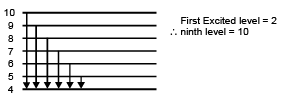Total line = 6

QUESTION: 21

The first use of quantum theory to explain the structure of atom was made by :

Solution:

Bohr's theory was based upon some postulations of classical physics and some postulations of the newly proposed quantum theory of Planck.

QUESTION: 22

The wavelength associated with a golf ball weighing 200g and moving at a speed of 5m/h is of the order:

Solution:

From de Broglie wavelength formula; λ = h/p where h=Planck's constant and p=momentum.
Putting all the values, we have h=6.626×10-34/(200/1000)(5/3600)
= 2.3853×10-30
Order is of 10-30

QUESTION: 23

The longest wavelength of He+ in Paschen series is "m", then shortest wavelength of Be3+ in Paschen series is (in terms of m) :

Solution:

The expression for the wavelength is :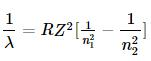For the longest wavelength of the He+ atom in Paschen series , the expression becomes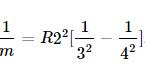(1)

For the shortest wavelength of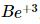the expression becomes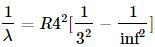(2)
Divide equation (2) with equation (1).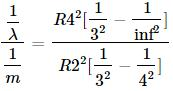On solving this, we get λ=7/64m

QUESTION: 24

What is uncertainity in location of a photon of wavelength 5000 Å if wavelength is known to an accuracy of 1 pm?

Solution: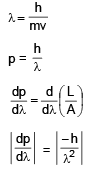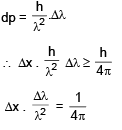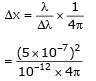0.0199 m

QUESTION: 25

Consider the following nuclear reactions involving X & Y.

X → Y + 2He4                      Y → 8O18 + 1H1

If both neutrons as well as protons in both the sides are conserved in nuclear reaction then moles of neutrons in 4.6 gm of X :

Solution: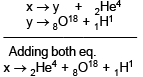By conservation of mass
X = 4 + 18 + 1 gm
= 23
23 gm → (2 + 10) moles neutrons.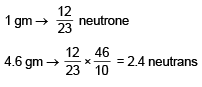QUESTION: 26

Electromagnetic radiations having λ = 310 Å are subjected to a metal sheet having work function = 12.8 eV. What will be the velocity of photoelectrons with maximum Kinetic energy.

Solution: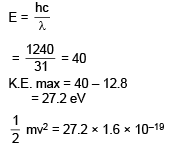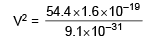V2 = 9.56 × 1012
V = 3.09 × 106 m/sec.

QUESTION: 27

Assuming Heisenberg Uncertainity Principle to be true what could be the miniumum uncertainity in de–Broglie wavelength of a moving electron accelerated by Potential Difference of 6V whose uncertainity in position is 7/22 n.m.

Solution: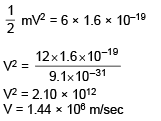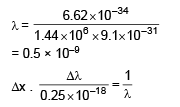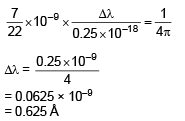QUESTION: 28

A 1-kW radio transmitter operates at a frequency of 880 Hz. How many photons per second does it emit -

Solution:

Given :
P = 1 kW
P = 1 × 103 watt
E = 103 J/S in one sec
v = 880 Hz
∴ E = nhv
⇒  103 x  ×  6.626 × 10–34 × 880
⇒  x = 1.71 × 1033 in one sec

QUESTION: 29

The value of Bohr radius of hydrogen atom is -

Solution: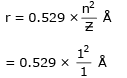= 0.529 × 10–10 m
= 0.529 × 10–8 cm

QUESTION: 30

On the basis of Bohr's model, the radius of the 3rd orbit is -

Solution: